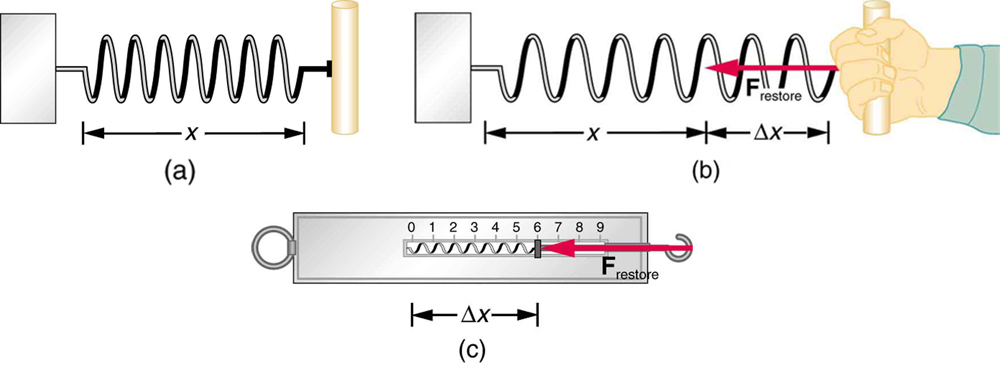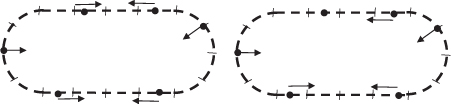# 4.1 Development of force concept  (Page 2/6)

 Page 2 / 6

A more quantitative definition of force can be based on some standard force, just as distance is measured in units relative to a standard distance. One possibility is to stretch a spring a certain fixed distance, as illustrated in [link] , and use the force it exerts to pull itself back to its relaxed shape—called a restoring force —as a standard. The magnitude of all other forces can be stated as multiples of this standard unit of force. Many other possibilities exist for standard forces. (One that we will encounter in Magnetism is the magnetic force between two wires carrying electric current.) Some alternative definitions of force will be given later in this chapter.The force exerted by a stretched spring can be used as a standard unit of force. (a) This spring has a length x size 12{x} {} when undistorted. (b) When stretched a distance Δ x size 12{Dx} {} , the spring exerts a restoring force, F restore size 12{F rSub { size 8{"restore"} } } {} , which is reproducible. (c) A spring scale is one device that uses a spring to measure force. The force F restore size 12{F rSub { size 8{"restore"} } } {} is exerted on whatever is attached to the hook. Here F restore size 12{F rSub { size 8{"restore"} } } {} has a magnitude of 6 units in the force standard being employed.

## Take-home experiment: force standards

To investigate force standards and cause and effect, get two identical rubber bands. Hang one rubber band vertically on a hook. Find a small household item that could be attached to the rubber band using a paper clip, and use this item as a weight to investigate the stretch of the rubber band. Measure the amount of stretch produced in the rubber band with one, two, and four of these (identical) items suspended from the rubber band. What is the relationship between the number of items and the amount of stretch? How large a stretch would you expect for the same number of items suspended from two rubber bands? What happens to the amount of stretch of the rubber band (with the weights attached) if the weights are also pushed to the side with a pencil?

## Test prep for ap courses

The figure above represents a racetrack with semicircular sections connected by straight sections. Each section has length d , and markers along the track are spaced d /4 apart. Two people drive cars counterclockwise around the track, as shown. Car X goes around the curves at constant speed v c, increases speed at constant acceleration for half of each straight section to reach a maximum speed of 2 v c, then brakes at constant acceleration for the other half of each straight section to return to speed v c. Car Y also goes around the curves at constant speed v c, increases its speed at constant acceleration for one-fourth of each straight section to reach the same maximum speed 2 v c, stays at that speed for half of each straight section, then brakes at constant acceleration for the remaining fourth of each straight section to return to speed v c.

(a) On the figures below, draw an arrow showing the direction of the net force on each of the cars at the positions noted by the dots. If the net force is zero at any position, label the dot with 0.

The position of the six dots on the Car Y track on the right are as follows:

• The first dot on the left center of the track is at the same position as it is on the Car X track.
• The second dot is just slight to the right of the Car X dot (less than a dash) past three perpendicular hash marks moving to the right.
• The third dot is about one and two-thirds perpendicular hash marks to the right of the center top perpendicular has mark.
• The fourth dot is in the same position as the Car X figure (one perpendicular hash mark above the center right perpendicular hash mark).
• The fifth dot is about one and two-third perpendicular hash marks to the right of the center bottom perpendicular hash mark.
• The sixth dot is in the same position as the Car Y dot (one and two third perpendicular hash marks to the left of the center bottom hash mark).

(b)

i. Indicate which car, if either, completes one trip around the track in less time, and justify your answer qualitatively without using equations.

ii. Justify your answer about which car, if either, completes one trip around the track in less time quantitatively with appropriate equations.Car X is shown on the left, and Car Y is shown on the right.

i.

Car X takes longer to accelerate and does not spend any time traveling at top speed. Car Y accelerates over a shorter time and spends time going at top speed. So Car Y must cover the straightaways in a shorter time. Curves take the same time, so Car Y must overall take a shorter time.

ii.

The only difference in the calculations for the time of one segment of linear acceleration is the difference in distances. That shows that Car X takes longer to accelerate. The equation $\frac{d}{4{v}_{c}}={t}_{c}$ corresponds to Car Y traveling for a time at top speed.

Substituting $a=\frac{{v}_{c}}{{t}_{1}}$ into the displacement equation in part (b) ii gives $D=\frac{3}{2}{v}_{c}{t}_{1}$ . This shows that a car takes less time to reach its maximum speed when it accelerates over a shorter distance. Therefore, Car Y reaches its maximum speed more quickly, and spends more time at its maximum speed than Car X does, as argued in part (b) i.

Which of the following is an example of a body exerting a force on itself?

1. a person standing up from a seated position
2. a car accelerating while driving
3. both of the above
4. none of the above

A hawk accelerates as it glides in the air. Does the force causing the acceleration come from the hawk itself? Explain.

A body cannot exert a force on itself. The hawk may accelerate as a result of several forces. The hawk may accelerate toward Earth as a result of the force due to gravity. The hawk may accelerate as a result of the additional force exerted on it by wind. The hawk may accelerate as a result of orienting its body to create less air resistance, thus increasing the net force forward.

What causes the force that moves a boat forward when someone rows it?

1. The force is caused by the rower’s arms.
2. The force is caused by an interaction between the oars and gravity.
3. The force is caused by an interaction between the oars and the water the boat is traveling in.
4. The force is caused by friction.

## Section summary

• Dynamics is the study of how forces affect the motion of objects.
• Force is a push or pull that can be defined in terms of various standards, and it is a vector having both magnitude and direction.
• External forces are any outside forces that act on a body. A free-body diagram    is a drawing of all external forces acting on a body.

## Conceptual questions

Propose a force standard different from the example of a stretched spring discussed in the text. Your standard must be capable of producing the same force repeatedly.

What properties do forces have that allow us to classify them as vectors?

If a prism is fully imersed in water then the ray of light will normally dispersed or their is any difference?
the same behavior thru the prism out or in water bud abbot
Ju
If this will experimented with a hollow(vaccum) prism in water then what will be result ?
Anurag
What was the previous far point of a patient who had laser correction that reduced the power of her eye by 7.00 D, producing a normal distant vision power of 50.0 D for her?
What is the far point of a person whose eyes have a relaxed power of 50.5 D?
Jaydie
What is the far point of a person whose eyes have a relaxed power of 50.5 D?
Jaydie
A young woman with normal distant vision has a 10.0% ability to accommodate (that is, increase) the power of her eyes. What is the closest object she can see clearly?
Jaydie
29/20 ? maybes
Ju
In what ways does physics affect the society both positively or negatively
how can I read physics...am finding it difficult to understand...pls help
try to read several books on phy don't just rely one. some authors explain better than other.
Ju
And don't forget to check out YouTube videos on the subject. Videos offer a different visual way to learn easier.
Ju
hope that helps
Ju
I have a exam on 12 february
what is velocity
Jiti
the speed of something in a given direction.
Ju
what is a magnitude in physics
Propose a force standard different from the example of a stretched spring discussed in the text. Your standard must be capable of producing the same force repeatedly.
What is meant by dielectric charge?
what happens to the size of charge if the dielectric is changed?
omega= omega not +alpha t derivation
u have to derivate it respected to time ...and as w is the angular velocity uu will relace it with "thita × time""
Abrar
do to be peaceful with any body
the angle subtended at the center of sphere of radius r in steradian is equal to 4 pi how?
if for diatonic gas Cv =5R/2 then gamma is equal to 7/5 how?
Saeed
define variable velocity
displacement in easy way.
binding energy per nucleonByByByByByBy